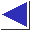# comp.lang.c FAQ list ·Question 20.8

Q: How can I implement sets or arrays of bits?

A: Use arrays of char or int, with a few macros to access the desired bit in the proper cell of the array. Here are some simple macros to use with arrays of char:

```#include <limits.h>		/* for CHAR_BIT */

#define BITMASK(b) (1 << ((b) % CHAR_BIT))
#define BITSLOT(b) ((b) / CHAR_BIT)
#define BITSET(a, b) ((a)[BITSLOT(b)] |= BITMASK(b))
#define BITCLEAR(a, b) ((a)[BITSLOT(b)] &= ~BITMASK(b))
#define BITTEST(a, b) ((a)[BITSLOT(b)] & BITMASK(b))
#define BITNSLOTS(nb) ((nb + CHAR_BIT - 1) / CHAR_BIT)
```
(If you don't have <limits.h>, try using 8 for CHAR_BIT.)

Here are some usage examples. To declare an ``array'' of 47 bits:

```	char bitarray[BITNSLOTS(47)];
```
To set the 23rd bit:
```	BITSET(bitarray, 23);
```
To test the 35th bit:
```	if(BITTEST(bitarray, 35)) ...
```
To compute the union of two bit arrays and place it in a third array (with all three arrays declared as above):
```	for(i = 0; i < BITNSLOTS(47); i++)
array3[i] = array1[i] | array2[i];
```
To compute the intersection, use & instead of |.

As a more realistic example, here is a quick implementation of the Sieve of Eratosthenes, for computing prime numbers:

```#include <stdio.h>
#include <string.h>

#define MAX 10000

int main()
{
char bitarray[BITNSLOTS(MAX)];
int i, j;

memset(bitarray, 0, BITNSLOTS(MAX));

for(i = 2; i < MAX; i++) {
if(!BITTEST(bitarray, i)) {
printf("%d\n", i);
for(j = i + i; j < MAX; j += i)
BITSET(bitarray, j);
}
}
return 0;
}
```